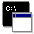## Firefly and PC GAMESS-related discussion club

Learn how to ask questions correctlyEnergy rises and oscillate at the end of optimization

Oleg Levitskiy
levicoleg@mail.ru

Dear Firefly Users,
I've tryed to perform an optimization of anionic complex of Ni (DFT/PBE96, PCM) and faced a problem with convergence. Energy has unexpectedly risen and fluctuate spontaneousely from step to step. I've tryed to increase accuracy (ICUT, ITOL values and also DLCTOL and ORTTOL), to change optimization method (GDIIS, NR), to perform optimization in cartesian or delocalized coordinates, but the result was similar in all cases. Could you advise me something else to solve this problem.
The beginning of my input is following:

\$contrl scftyp=rhf dfttyp=pbe96
runtyp=OPTIMIZE icharg=-1 nprint=-5
maxit=100 ICUT=20 ITOL=30 NZVAR=168 \$end
\$system kdiag=-1 mwords=100 \$end
\$SCF DIRSCF=.true. maxdii=20 \$end
\$STATPT method=NR \$end
\$ZMAT DLC=.T. AUTO=.T.
DLCTOL=1D-7 ORTTOL=1D-7 \$end
\$INTGRL PACKAO=.true. \$end
\$p2p p2p=.t. dlb=.t. \$end
\$basis gbasis=TZV \$end
\$PCM PCMTYP=DPCM SOLVNT=input RSOLV=2.155 EPS=35.688 EPSINF=1.806874 \$END
\$PCMCAV RIN(58)=1.956 \$END

Typical out-file is attached.

Thank you,

OlegThis message contains the 5505 kb attachment [ GlyNi_deprot_MeCN_2.out ]Tue Sep 9 '14 3:30pmThis message read 637 times